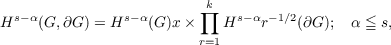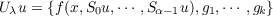#### Vol. 26, No. 2, 1968

 Download this articleFor screen For printingRecent Issues Vol. 325: 1 Vol. 324: 1  2 Vol. 323: 1  2 Vol. 322: 1  2 Vol. 321: 1  2 Vol. 320: 1  2 Vol. 319: 1  2 Vol. 318: 1  2Online Archive Volume: Issue:The Journal Subscriptions Editorial Board Officers Contacts Submission Guidelines Submission Form Policies for Authors ISSN: 1945-5844 (e-only) ISSN: 0030-8730 (print) Special Issues Author Index To Appear Other MSP Journals
Boundary value problems for elliptic convolution equations of Wiener-Hopf type in a bounded region

### Bui An Ton

Vol. 26 (1968), No. 2, 395–413
##### Abstract

Let A be an elliptic convolution operator of order α on a bounded open set G of Rn, α > 0. Let Aj be the principal part of A in a local coordinates system and Ãj(xj) be its symbol with a Wiener-Hopf type of factorization with respect to ξn : Ãj(xj) = Ãj+(xj)Aj(xj) for xnj = 0. Aj+ is analytic in Im ξn > 0, is homogeneous of order k in ξ, k is a positive integer, k < α. Ãj is analytic in Im ξn 0. Let Br;r = 1,,k be a system of convolution operators on ∂G, of orders αr;0 αr < α and let Brj be the principal part of Br in a local coordinates system. The Ãj+, Brj are assumed to satisfy a Shapiro-Lopatinskii type of condition for each j.

Visik and Eskin have shown that the operator U from H+s(G) intodefined by: Uu = {Au,B1u,,Bku} is of Fredholm type. In this paper, we show the smoothness in the interior of the solutions of Uu = (f,g1,,gk). We prove that if Ãj+, Brj satisfy a strengthened form of the Shapiro-Lopatinskii condition, then the operator Uλu = {(A + λα)u,B1u,,Bku} is one-to-one and onto. The nonlinear problem:has a solution in H+α(G). f(x,ζ0,α1) is continuous in all the variables and has at most a linear growth in (ζ0,α1). If the set Ω = {u : u H+α(G),Bru = 0 on ∂G,r = 1,,k} is dense in L2(G), then the completeness in L2(G) of the generalized eigenfunctions of the operator A2 associated with Uu = {f,0,,0} is established.

Primary: 35J99
Secondary: 35S15
##### Milestones